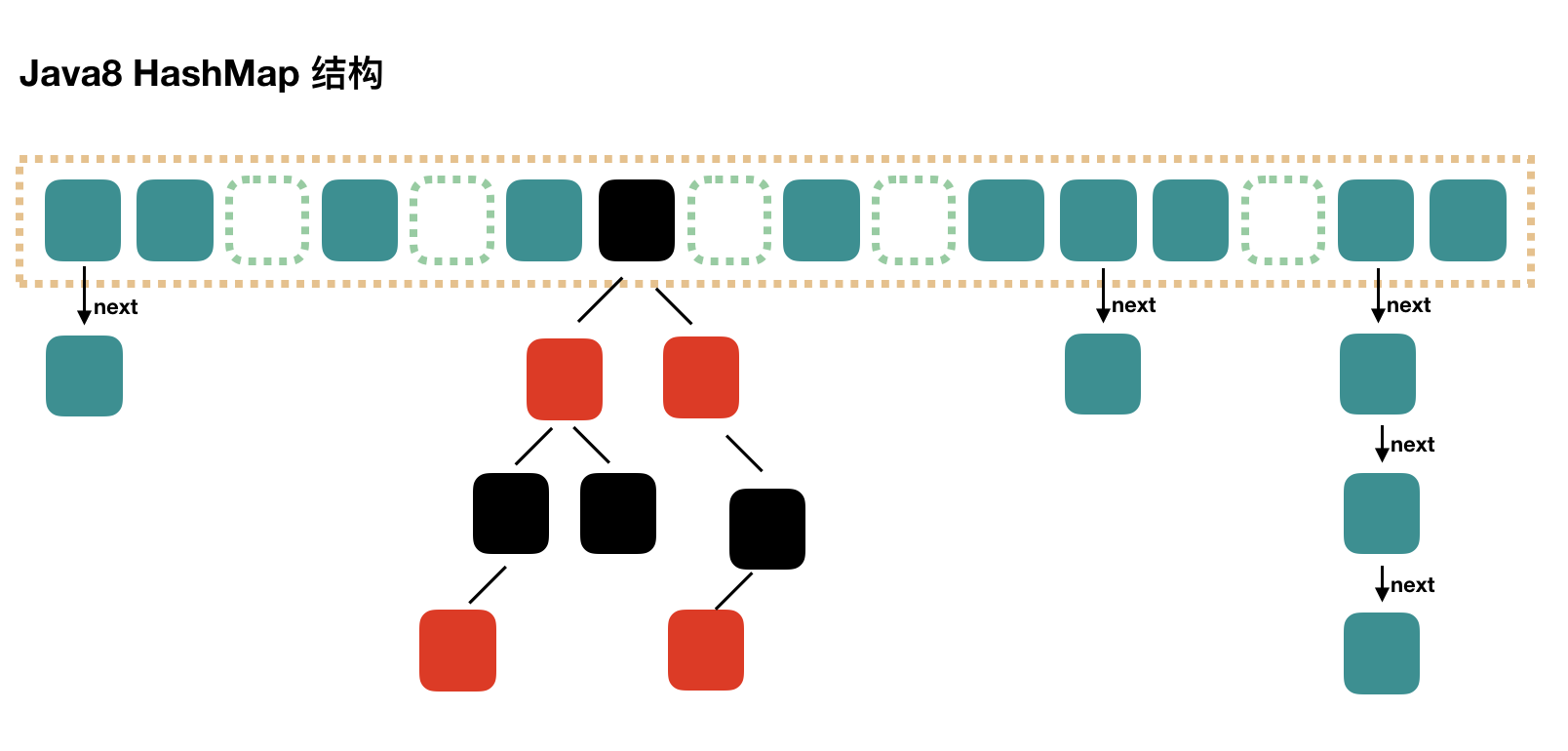# HashMap源码详解

HashMap是Java开发中常用的一种数据接口，常用于完成key:value结构的存储。而同时，HashMap又是HashSet、HashTable、ConcurrentHashMap这三种数据结构的基础。

# 1 基本结构

HashMap使我们非常常用的一种数据结构，常用来存储各种键值数据。同时，它也是最为简单的。这里我们的讲解以Java 1.8 为例。

HashMap的结构示意图如下：## 1.1 动态数组

• capacity：目前数组的长度。为了实现高效的扩容，其值总为2^n的形式。每次扩容后，n会加1，即整个数组的容量变为之前的2倍。该值初始默认值为16。

## 1.2 数组元素

• hash: 当前位置值的hash值
• key：当前位置的键
• value: 当前位置存储的值
• next;下一个Node

# 2 初始化操作

public HashMap(int initialCapacity, float loadFactor) {
if (initialCapacity < 0)
throw new IllegalArgumentException("Illegal initial capacity: " +
initialCapacity);
if (initialCapacity > MAXIMUM_CAPACITY)
initialCapacity = MAXIMUM_CAPACITY;
throw new IllegalArgumentException("Illegal load factor: " +
this.threshold = tableSizeFor(initialCapacity);
}


# 3 数据写入操作

• 计算要插入数据的Hash值，并根据该值确定元素的插入位置（即在动态数组中的位置）。
• 将元素放入到数组的指定位置
• 如果该数组位置之前没有元素，则直接放入
• 放入该位置后，数组元素超过扩容阈值，则对数组进行扩容
• 放入该位置后，数组元素没超过扩容阈值，写入结束
• 如果该数组位置之前有元素，则挂载到已有元素的后端
• 如果之前元素组成了树，则挂入树的指定位置
• 如果之前元素组成了链表
• 如果加入该元素链表长度超过8，则将链表转化为红黑树后插入
• 如果加入该元素链表长度不超过8，则直接插入

// 供外部调用的方法
public V put(K key, V value) {
return putVal(hash(key), key, value, false, true);
}

final V putVal(int hash, K key, V value, boolean onlyIfAbsent,
boolean evict) {
Node<K,V>[] tab; Node<K,V> p; int n, i;
// 这里如果发现动态数组为null则会初始化数组。因此第一次放入值时会在这里初始化数组
if ((tab = table) == null || (n = tab.length) == 0)
n = (tab = resize()).length;
// 通过hash发现要放入的元素的数组位置为null，则直接把该元素放在这里即可
if ((p = tab[i = (n - 1) & hash]) == null)
tab[i] = newNode(hash, key, value, null);
else {
// 如果要放入的位置已经有元素了
Node<K,V> e; K k;
// 判断原位置第一个元素是否和新元素key完全一致
if (p.hash == hash &&
((k = p.key) == key || (key != null && key.equals(k))))
e = p;
else if (p instanceof TreeNode)
// 说明是红黑树，按照红黑树方法放入新节点
e = ((TreeNode<K,V>)p).putTreeVal(this, tab, hash, key, value);
else {
// 说明是列表，按照列表方法放入新节点
for (int binCount = 0; ; ++binCount) {
if ((e = p.next) == null) {
p.next = newNode(hash, key, value, null);
if (binCount >= TREEIFY_THRESHOLD - 1) // -1 for 1st
// 如果放入列表后列表过长，则将列表转为红黑树
treeifyBin(tab, hash);
break;
}
if (e.hash == hash &&
((k = e.key) == key || (key != null && key.equals(k))))
break;
p = e;
}
}

// 这里说明新值和旧值的key完全相同，进行覆盖操作
if (e != null) { // existing mapping for key
V oldValue = e.value;
if (!onlyIfAbsent || oldValue == null)
e.value = value;
afterNodeAccess(e);
return oldValue;
}
}
++modCount;
if (++size > threshold)
resize();
afterNodeInsertion(evict);
return null;
}


# 4 数据读取操作

• 根据要取得key的值，hash出数组中的指定位置
• 取出指定位置的元素（这时，key的hash值是一样的）
• 如果key也完全一样，则返回该值，查找结束。
• 如果key不一样，判断其后面挂载的是树还是列表
• 如果是树，按照树的方法查找
• 如果是列表，按照列表的方法查找

// 供外部调用的方法
public V get(Object key) {
Node<K,V> e;
return (e = getNode(hash(key), key)) == null ? null : e.value;
}

final Node<K,V> getNode(int hash, Object key) {
Node<K,V>[] tab; Node<K,V> first, e; int n; K k;
// 判断数组存在且不为空，否则直接返回null
if ((tab = table) != null && (n = tab.length) > 0 &&
(first = tab[(n - 1) & hash]) != null) {
if (first.hash == hash && // always check first node
// 第一个节点key与要查找的完全一致
((k = first.key) == key || (key != null && key.equals(k))))
return first;
if ((e = first.next) != null) {
// 第一个节点key与要查找的不一致
if (first instanceof TreeNode)
// 按照树的方法查找
return ((TreeNode<K,V>)first).getTreeNode(hash, key);
do {
// 按照列表方法查找
if (e.hash == hash &&
((k = e.key) == key || (key != null && key.equals(k))))
return e;
} while ((e = e.next) != null);
}
}
return null;
}


# 5 扩容操作

• 一是将数组的长度变为原来的两倍。
• 二是将已经hash分布到数组中的所有元素重新计算hash值，分配到新的数组中。

## 5.1 哈希与重哈希

static final int hash(Object key) {
int h;
return (key == null) ? 0 : (h = key.hashCode()) ^ (h >>> 16);
}


tab[i = (n - 1) & hash]


(n-1)为数组中的最大位置，hash为哈希的结果，两者进行了逻辑与操作。我们到二进制中去理解，那逻辑与操作我们可以理解为hash值的二进制数在(n-1)的二进制数上的求交集操作，我们即为p。最终得到的结果肯定小于等于(n-1)。

## 5.2 扩容

final Node<K,V>[] resize() {
Node<K,V>[] oldTab = table;
int oldCap = (oldTab == null) ? 0 : oldTab.length;
int oldThr = threshold;
int newCap, newThr = 0;
if (oldCap > 0) {
if (oldCap >= MAXIMUM_CAPACITY) {
// 如果长度过长，则不进行扩展
threshold = Integer.MAX_VALUE;
return oldTab;
}
else if ((newCap = oldCap << 1) < MAXIMUM_CAPACITY &&
oldCap >= DEFAULT_INITIAL_CAPACITY)
// 否则，新长度是原来的两倍
newThr = oldThr << 1; // double threshold
}
else if (oldThr > 0) // initial capacity was placed in threshold
newCap = oldThr;
else {               // zero initial threshold signifies using defaults
newCap = DEFAULT_INITIAL_CAPACITY;
}
if (newThr == 0) {
float ft = (float)newCap * loadFactor;
newThr = (newCap < MAXIMUM_CAPACITY && ft < (float)MAXIMUM_CAPACITY ?
(int)ft : Integer.MAX_VALUE);
}
threshold = newThr;
// 创建一个新的数组
@SuppressWarnings({"rawtypes","unchecked"})
Node<K,V>[] newTab = (Node<K,V>[])new Node[newCap];
table = newTab;
if (oldTab != null) {
// 轮询操作，对所有元素重哈希
for (int j = 0; j < oldCap; ++j) {
Node<K,V> e;
if ((e = oldTab[j]) != null) {
oldTab[j] = null;
if (e.next == null)
newTab[e.hash & (newCap - 1)] = e;
else if (e instanceof TreeNode)
// 树元素重hash
((TreeNode<K,V>)e).split(this, newTab, j, oldCap);
else { // preserve order
// 略：链表元素重hash
}
}
}
}
return newTab;
}


# 6 总结

HashMap是Java中常用的类，本文HashMap的结构和源码进行了详细的梳理和分析。包括HashMap的数据结构、数据存取、数据写入、扩容等方法。